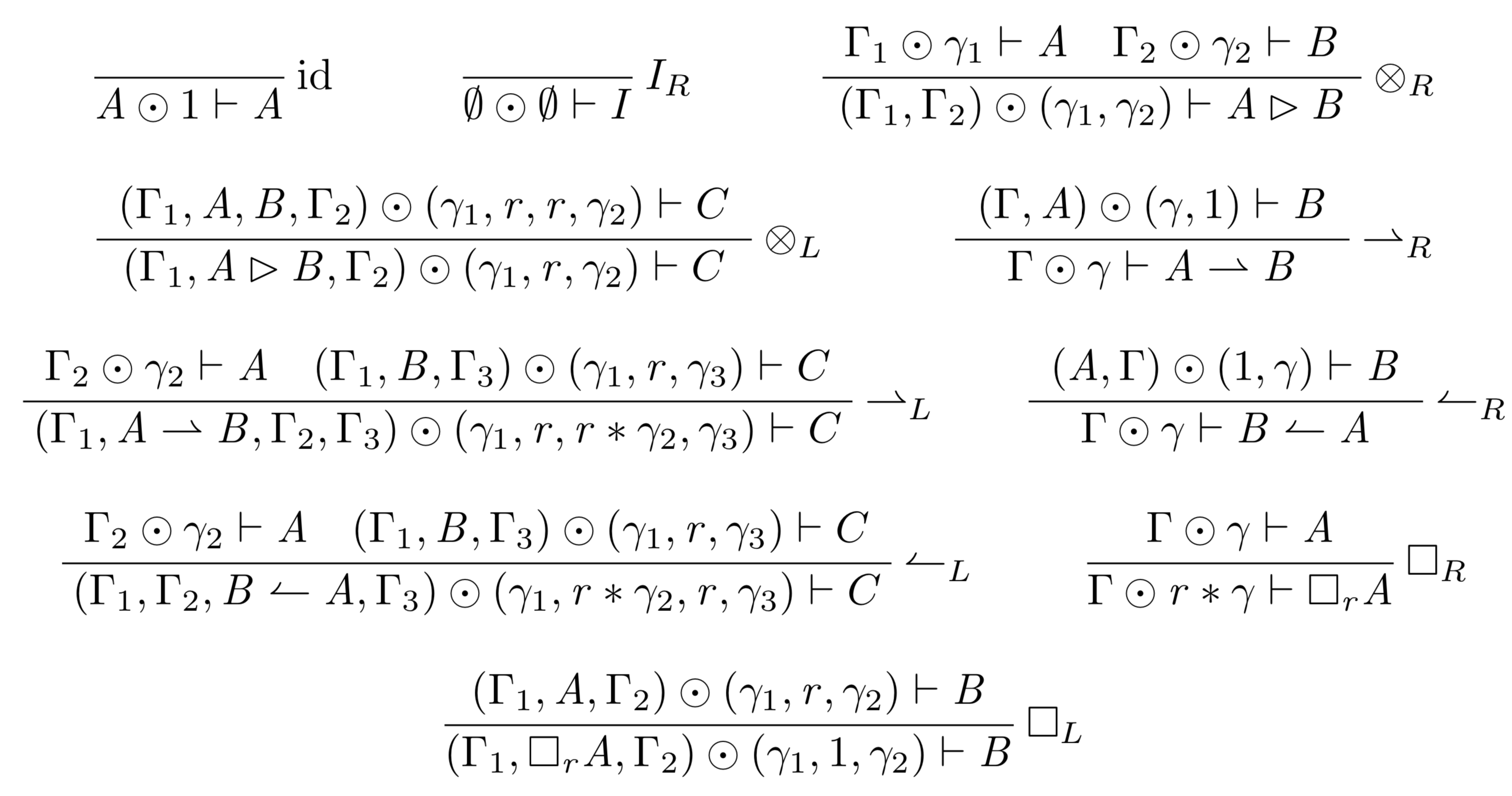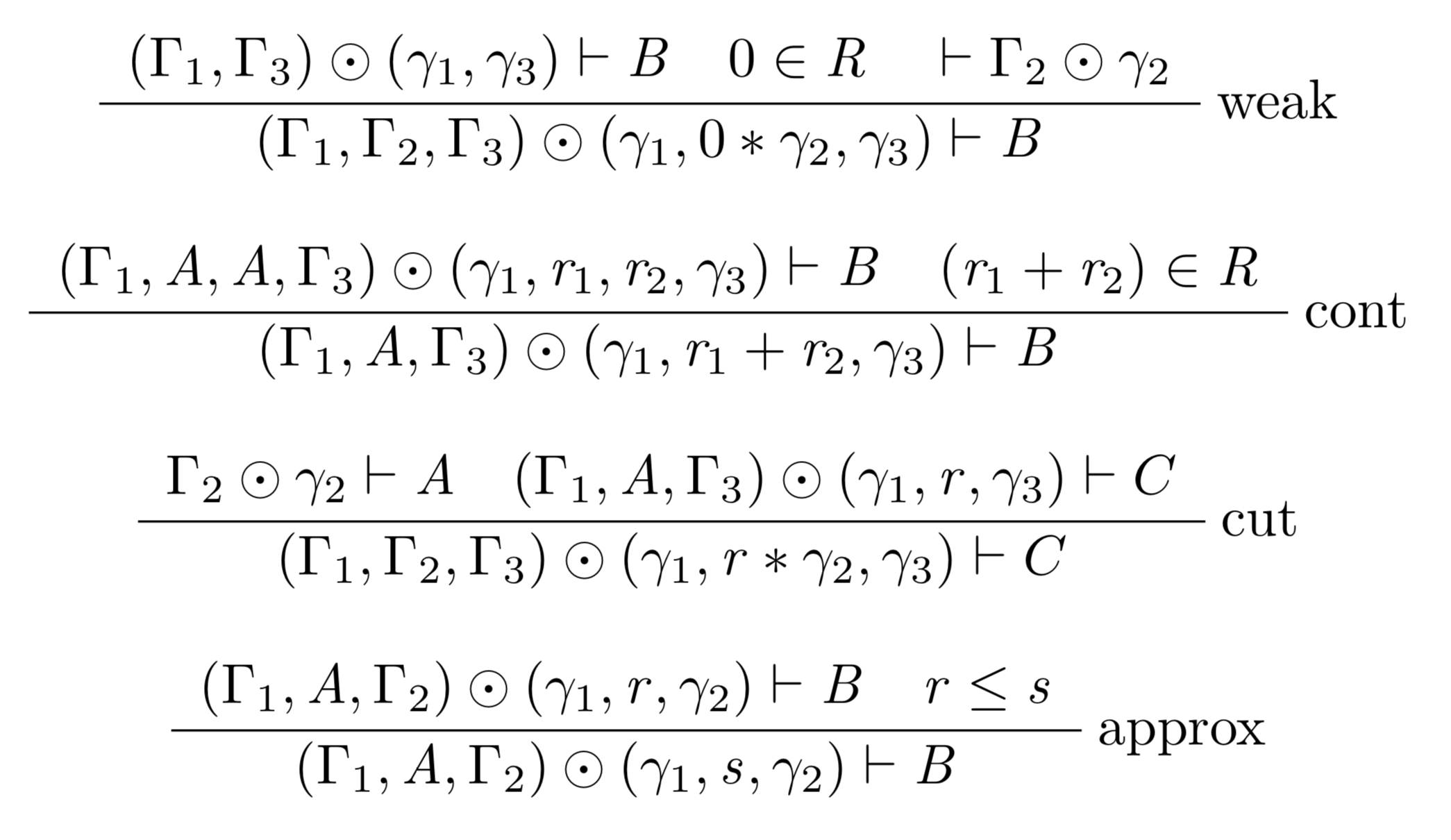## Units in Graded Sequent Calculi

Updated: 2020-03-25

This is a guest post by Aubrey Bryant.

If you are searching for a logical system as exact and careful with resources as that infamous miser, Ebenezer Scrooge, look no further. Graded modal linear logic is the system for you. Like linear logic, it is resource-sensitive; however, it goes a step further and tracks not just binary usage, but usage along a continuum of grades, specified by a graded context. The grades can be defined in many different ways, but for simplicity while introducing the concept, let’s imagine that the grades range over the natural numbers, and indicate the number of times a formula must be used. When a formula $A$ is needed at a grade of $r$ to yield a consequent $B$, the grade for $A$ must be added to the account for $B$, once for each use of $A$ required to yield $B$. Thus, $A$’s grade must be multiplied by $r$ in order to yield the required number of $A$’s. To illustrate, here is the cut rule in our graded system: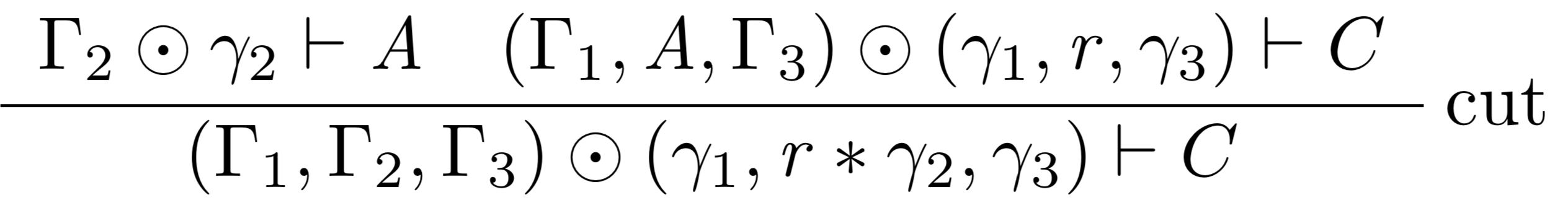$\Gamma_1 \odot \gamma_1$ is the context yielding one $A$, where $\gamma_1$ is a vector containing a grade for every corresponding element of $\Gamma_1$; the grade describes the usage for each resource in the context. In our current example, it indicates the number of times that resource must be used. Note that $\gamma_1$ is multiplied by $r$ to yield the required number of $A$’s.

Here is a very simple example that illustrates how the grading works. In this derivation, $A \rhd A$ is only derivable when there are two $A$’s available to use: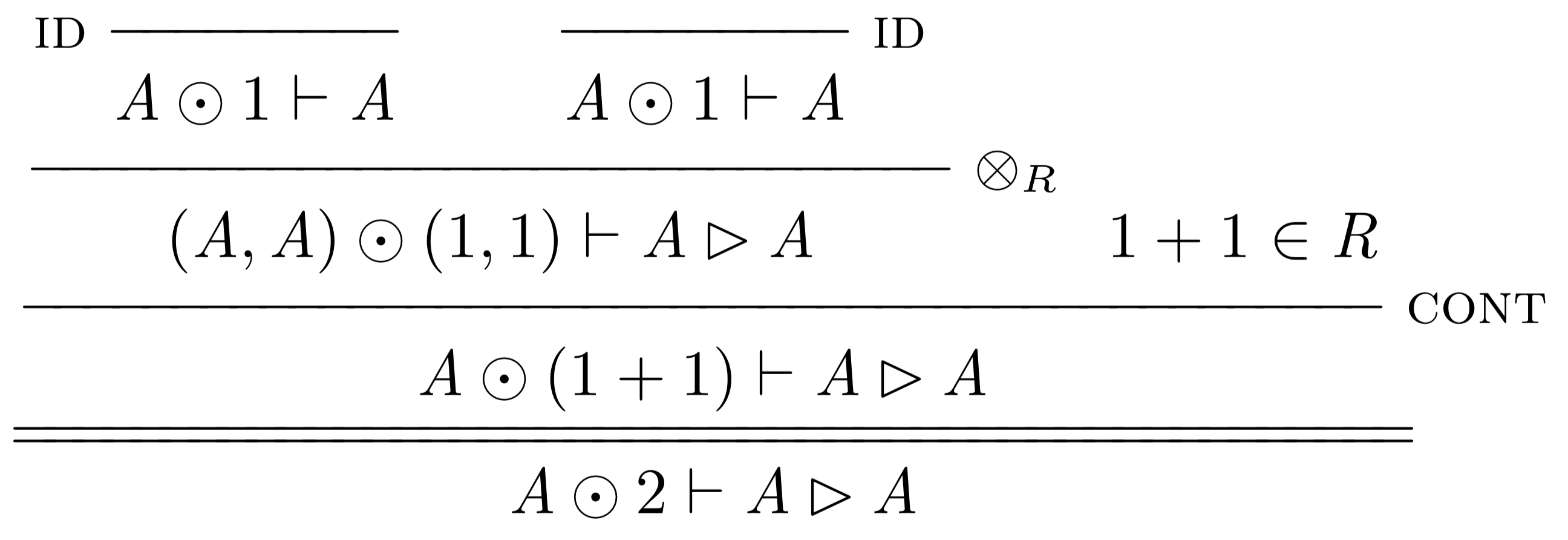While this watchful precision can be somewhat uncomfortable if you’re Bob Cratchitt asking for an extra lump of coal for the stove, remember that Scrooge became a wealthy man through such careful accounting. And, literary references aside, I actually appreciate the subtlety and precision that this system requires. I have been working through a cut-elimination proof for this system for the past couple of months, following the blueprint laid out by Melliès. I started with what I thought were the juiciest and most interesting steps, like cuts with tensor products and linear implication playing primary or secondary roles. Then I turned to what I thought would be the boring bits, like those involving the axiom of identity and the tensor unit rules. I expected these to be pretty rote - after all, they are specifically designed to do nothing. But in a system that keeps such careful account, doing nothing is surprisingly difficult. In fact, under the first system of rules, the left rule for tensor unit introduction caused cut-elimination to fail.

Our initial formulation of the left rule for the tensor unit was: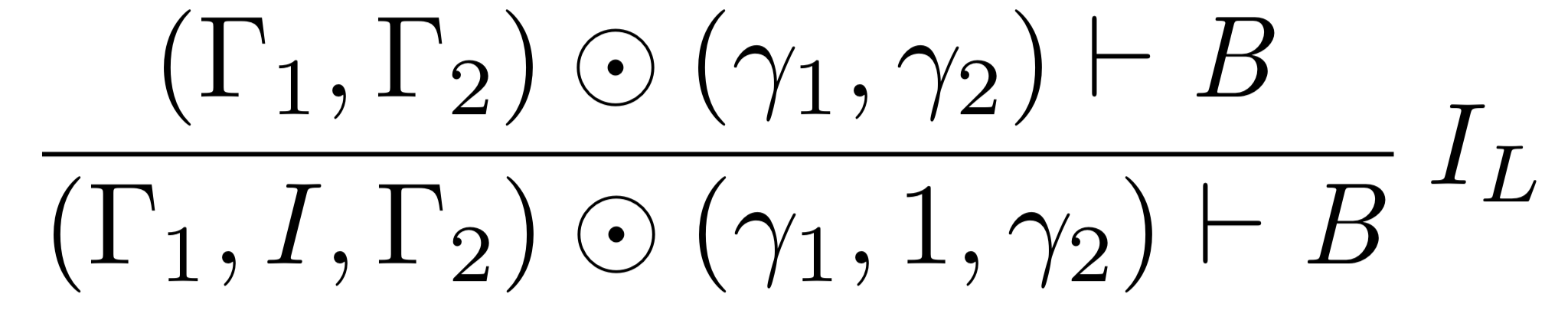Notice that this rule allows you to introduce $I$ into the context, at a grade of $1$. In ordinary linear logic, the tensor unit is sometimes called the ‘empty resource’, unique among linear hypotheses in that it is the only one derivable from the empty context, and similarly the only one that can be discarded without being used. This behavior is a clue that the grade of $1$ is incorrect, since anything with a nonzero grade makes an impact on our ‘bill’, in the language of Scrooge. And certainly, the careful accounting demanded by our system made a vivid case for why the unit grade was wrong.

Here is an illustration of the problem, using cut (defined above) and contraction, defined as: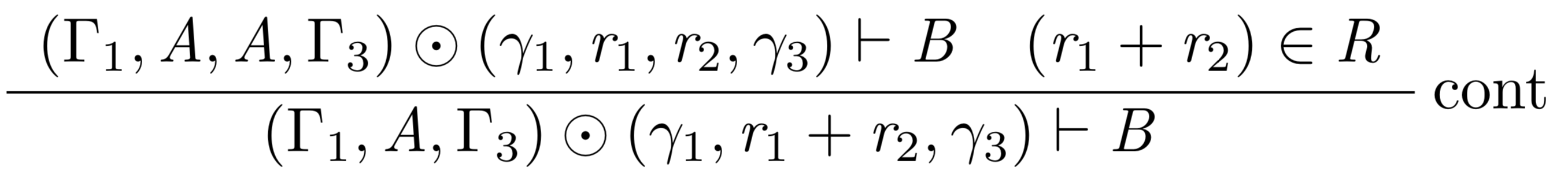Following the cut-elimination procedure outlined by Melliès, the general strategy is to show how every complex cut can be transformed into a simpler cut (or multiple simpler cuts). Applied repeatedly, this process moves the application of the cut rule into simpler and simpler proof steps until it is shown to be unnecessary at the axiom steps. So, in this case the task is to move the application of the cut rule to before the application of the left rule for the unit, without changing the final conclusion of the proof. For the case where the cut step follows left introduction of the tensor unit, the following proof transformation is required: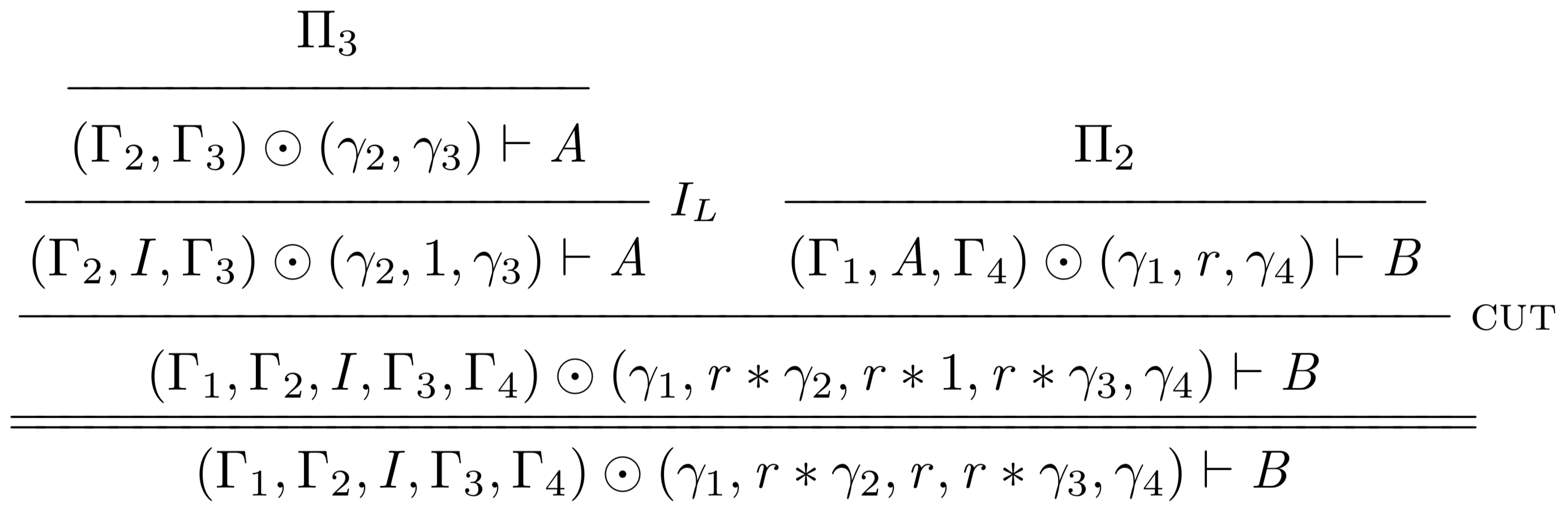becomes: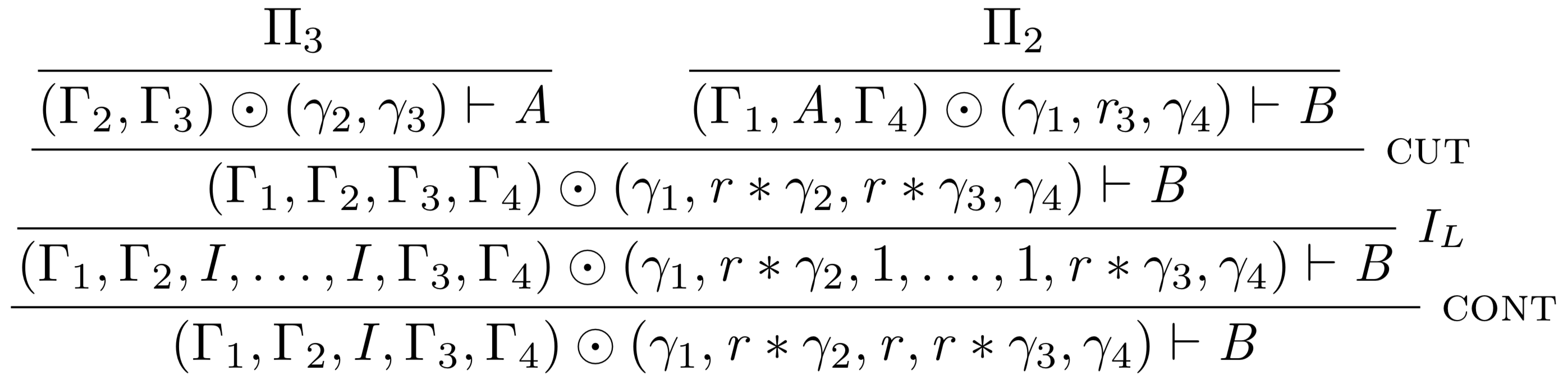To match the conclusion of the first proof, $I$ must have a grade of $r$ in the conclusion of the second proof. The proof above accomplishes this by doing $r$ applications of the left unit rule, and then subsequently $r-1$ applications of the contraction rule. This works for any $r > 0$, but not for the case when $r = 0$. Furthermore, this solution fails entirely when addition is not defined in the system, or when $1 + 1 \notin \mathcal{R}$, as this is the only option available to match the grading.

The root of the problem is that $I$ gets a grade of $1$ in the left unit rule specified above. This means that when it is part of a context brought across a cut, it is multiplied by the required grade $r$, and $1 * r = r$. Besides breaking cut elimination, grading $I$ with $1$ does not accurately capture the behavior we want, because we want to be able to ‘throw it away’ or leave it out of the grade accounting so we can use the tensor identity axioms:

$\begin{array}{l} A \rhd I \rightarrow A\\ I \rhd A \rightarrow A \end{array}$

With $I$ at a grade of $1$, we aren’t allowed to toss it out and keep only the $A$. While $1$ is a tempting grade to use, being the multiplicative unit, it does not produce the behavior we expect from $I$. The best option is to grade it at $0$ in its left rule, but then the rule is equivalent to weakening, and so we just let weakening take on both roles and throw out the left rule for the tensor unit altogether. This gives us the control we want while still allowing us to use the tensor identity axioms when weakening is allowed - that is, whenever $0 \in \mathcal{R}$. The new system has just a single right rule for tensor unit, and we have already proven cut elimination for it.

Checkout out new system the Graded Lambek Calculus below: